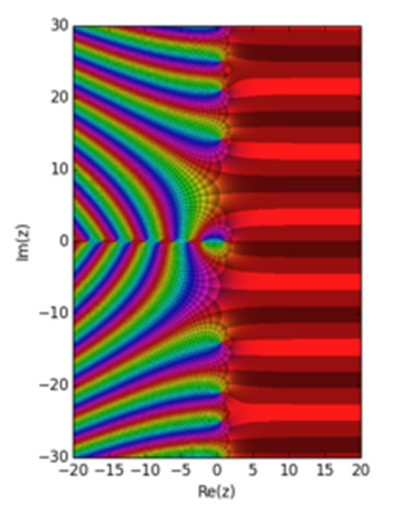# The Basel ProblemThe Rieman Zeta Function Template:Math represented in a rectangular region of the complex plane. It is generated as a matplotlib plot using a version of the Domain coloring methodEmpetrisor [CC BY-SA]One sometimes gets very interesting results when one turns divergent sums upside down.

The Basel problem was asked by Pietro Mengoli in $$1644$$.

He asked what is the exact summation of the reciprocals of the square numbers?

That is :

$$\displaystyle \sum_{n=1}^∞ \frac {1}{n^2} = \frac 11 + \frac 14 + \frac 19 + \frac {1}{16} +\frac {1}{25} + … = \,?$$

The approximate answer converges very slowly to about $$1.644934$$ but what is the exact value?

We start by asking an easier question.  What is the exact summation of the reciprocals of the pronic numbers?  Remember that the pronic numbers are the sums of the even numbers.

That is :

$$\displaystyle \sum_{k=1}^n 2k = n(n + 1)$$

Hence, the sum of the reciprocals of the pronics  is :

$$\displaystyle \sum_{n=1}^∞ \frac {1}{n(n + 1)} = \frac 12 + \frac 16 + \frac {1}{12} + \frac {1}{20} + \frac{1}{30} + …$$

Now we can add these terms to obtain partial sums :

$$\displaystyle \frac 12 + \frac 16 = \frac 23, \, \frac23 + \frac {1}{12} = \frac 34, \, \frac34 + \frac {1}{20} = \frac 45, \, \frac 45 + \frac {1}{30} = \frac 56$$

This is not a proof.  We can see the limit approaching one.  The proof relies on the fact that we can rewrite the terms and telescope them.

$$\displaystyle \frac 12 + \frac 16 + \frac {1}{12} + \frac {1}{20} + \frac {1}{30} + …$$

can be rewritten as :

$$\displaystyle \Bigl(1-\frac 12 \Bigr) + \Bigl(\frac12 – \frac 13\Bigr) + \Bigl(\frac 13 – \frac14\Bigr) + \Bigl(\frac 14 – \frac 15\Bigr) + \Bigl(\frac 15 – \frac 16\Bigr)+ …$$

which can be rewritten as :

$$\displaystyle 1 + \Bigl(-\frac12 + \frac12\Bigr) + \Bigl(-\frac 13 + \frac13\bigr) + \Bigl(-\frac 14 + \frac 14\Bigr) + \Bigl(-\frac 15 + \frac15\Bigr) \, – \frac 16 + …$$

The terms in the middle telescope and we are left with the first term and the last term.  As $$n$$ gets larger and larger the term $$\frac {1}{n(n + 1)}$$ gets smaller and smaller and approaches zero and the sum approaches the limit of one.

Hence, the sum of the reciprocals of the pronic numbers is :

$$\displaystyle \sum_{n=1}^∞ \frac {1}{n(n + 1)} = 1$$

A similar argument is made for the sum of the reciprocals of the triangular numbers (the sums of the counting numbers).

$$\displaystyle \sum_{k=1}^n k = \frac {n(n + 1)}{2}$$

Then the sum of the reciprocals of the triangular numbers is :

$$\displaystyle \sum_{n=1}^∞ \frac {2}{n(n + 1)} = 2\sum_{n=1}^∞ \frac {1}{n(n + 1)} = 2$$

Euler answered Mengoli’s question in 1735 and gave a more rigorous proof in 1741.

The sum of the odd numbers is the square numbers :

$$\displaystyle \sum_{k=1}^n 2k-1 = n^2$$

The sum of the reciprocals of the square numbers is :

$$\displaystyle \sum_{n=1}^∞ \frac {1}{n^2} = \frac {\pi^2}{6}$$

The maths is here for anyone that is interested.

An infinite series of surprises

The odd numbers always seem to do odd things.

Euler’s solution to the Basel problem is a special case of Riemann’s zeta function (also known as the Euler-Riemann zeta function) :

$$\displaystyle \zeta (s) = \sum_{n=1}^∞ \frac{1}{n^s}$$

where $$s$$ is a complex variable.

So

$$\zeta (2) = \frac {\pi^2}{6}$$

© OldTrout $$2019$$